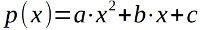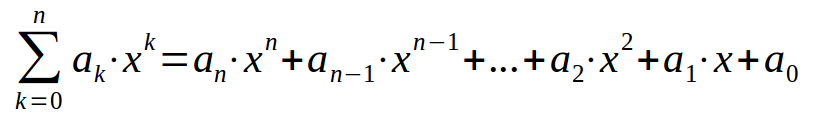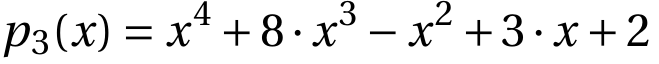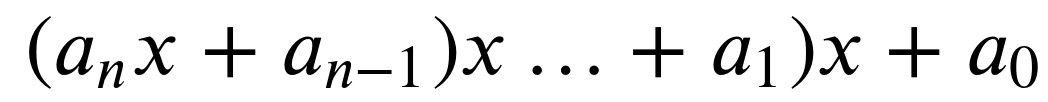## Decorators

### IntroductionDecorators belong most probably to the most beautiful and most powerful design possibilities in Python, but at the same time the concept is considered by many as complicated to get into. To be precise, the usage of decorators is very easy, but writing decorators can be complicated, especially if you are not experienced with decorators and some functional programming concepts.

Even though it is the same underlying concept, we have two different kinds of decorators in Python:

• Function decorators
• Class decorators

A decorator in Python is any callable Python object that is used to modify a function or a class. A reference to a function "func" or a class "C" is passed to a decorator and the decorator returns a modified function or class. The modified functions or classes usually contain calls to the original function "func" or class "C".

You may also consult our chapter on memoization with decorators.

If you like the image on the right side of this page and if you are also interested in image processing with Python, Numpy, Scipy and Matplotlib, you will definitely like our chapter on Image Processing Techniques, it explains the whole process of the making-of of our decorator and at sign picture!

### First Steps to Decorators

We know from our various Python training classes that there are some points in the definitions of decorators, where many beginners get stuck.

Therefore, we will introduce decorators by repeating some important aspects of functions. First you have to know or remember that function names are references to functions and that we can assign multiple names to the same function:

def succ(x):
return x + 1
successor = succ
successor(10)

Output: :
11
succ(10)

Output: :
11

This means that we have two names, i.e. "succ" and "successor" for the same function. The next important fact is that we can delete either "succ" or "successor" without deleting the function itself.

del succ
successor(10)

Output: :
11

#### Functions inside Functions

The concept of having or defining functions inside of a function is completely new to C or C++ programmers:

def f():

def g():
print("Hi, it's me 'g'")
print("Thanks for calling me")

print("This is the function 'f'")
print("I am calling 'g' now:")
g()

f()

This is the function 'f'
I am calling 'g' now:
Hi, it's me 'g'
Thanks for calling me


Another example using "proper" return statements in the functions:

def temperature(t):
def celsius2fahrenheit(x):
return 9 * x / 5 + 32

result = "It's " + str(celsius2fahrenheit(t)) + " degrees!"
return result

print(temperature(20))

It's 68.0 degrees!


#### Functions as Parameters

If you solely look at the previous examples, this doesn't seem to be very useful. It gets useful in combination with two further powerful possibilities of Python functions. Due to the fact that every parameter of a function is a reference to an object and functions are objects as well, we can pass functions - or better "references to functions" - as parameters to a function. We will demonstrate this in the next simple example:

def g():
print("Hi, it's me 'g'")
print("Thanks for calling me")

def f(func):
print("Hi, it's me 'f'")
print("I will call 'func' now")
func()

f(g)

Hi, it's me 'f'
I will call 'func' now
Hi, it's me 'g'
Thanks for calling me


You may not be satisfied with the output. 'f' should write that it calls 'g' and not 'func'. Of course, we need to know what the 'real' name of func is. For this purpose, we can use the attribute __name__, as it contains this name:

def g():
print("Hi, it's me 'g'")
print("Thanks for calling me")

def f(func):
print("Hi, it's me 'f'")
print("I will call 'func' now")
func()
print("func's real name is " + func.__name__)

f(g)

Hi, it's me 'f'
I will call 'func' now
Hi, it's me 'g'
Thanks for calling me
func's real name is g


The output explains what's going on once more. Another example:

import math

def foo(func):
print("The function " + func.__name__ + " was passed to foo")
res = 0
for x in [1, 2, 2.5]:
res += func(x)
return res

print(foo(math.sin))
print(foo(math.cos))

The function sin was passed to foo
2.3492405557375347
The function cos was passed to foo
-0.6769881462259364


#### Functions returning Functions

The output of a function is also a reference to an object. Therefore functions can return references to function objects.

def f(x):
def g(y):
return y + x + 3
return g

nf1 = f(1)
nf2 = f(3)

print(nf1(1))
print(nf2(1))

5
7


The previous example looks very artificial and absolutely useless. We will present now another language oriented example, which shows a more practical touch. Okay, still not a function which is useful the way it is. We write a function with the nearly self-explanatory name greeting_func_gen. So this function returns (or generates) functions which can be used to create people in different languages, i.e. German, French, Italian, Turkish, and Greek:

def greeting_func_gen(lang):
def customized_greeting(name):
if lang == "de":   # German
phrase = "Guten Morgen "
elif lang == "fr": # French
phrase = "Bonjour "
elif lang == "it": # Italian
phrase = "Buongiorno "
elif lang == "tr": # Turkish
phrase = "Günaydın "
elif lang == "gr": # Greek
phrase = "Καλημερα "
else:
phrase = "Hi "
return phrase + name + "!"
return customized_greeting

say_hi = greeting_func_gen("tr")
print(say_hi("Gülay"))    # this Turkish name means "rose moon" by the way

Günaydın Gülay!


It is getting more useful and at the same time more mathematically oriented in the following example. We will implement a polynomial "factory" function now. We will start with writing a version which can create polynomials of degree 2.The Python implementation as a polynomial factory function can be written like this:

def polynomial_creator(a, b, c):
def polynomial(x):
return a * x**2 + b * x + c
return polynomial

p1 = polynomial_creator(2, 3, -1)
p2 = polynomial_creator(-1, 2, 1)

for x in range(-2, 2, 1):
print(x, p1(x), p2(x))

-2 1 -7
-1 -2 -2
0 -1 1
1 4 2


We can generalize our factory function so that it can work for polynomials of arbitrary degree:def polynomial_creator(*coefficients):
""" coefficients are in the form a_n, ... a_1, a_0
"""
def polynomial(x):
res = 0
for index, coeff in enumerate(coefficients[::-1]):
res += coeff * x** index
return res
return polynomial

p1 = polynomial_creator(4)
p2 = polynomial_creator(2, 4)
p3 = polynomial_creator(1, 8, -1, 3, 2)
p4  = polynomial_creator(-1, 2, 1)

for x in range(-2, 2, 1):
print(x, p1(x), p2(x), p3(x), p4(x))

-2 4 0 -56 -7
-1 4 2 -9 -2
0 4 4 2 1
1 4 6 13 2


The function p3 implements, for example, the following polynomial:The polynomial function inside of our decorator polynomial_creator can be implemented more efficiently. We can factorize it in a way so that it doesn't need any exponentiation.

Factorized version of a general polynomial without exponentiation:Implementation of our polynomial creator decorator avoiding exponentiation:

def polynomial_creator(*coeffs):
""" coefficients are in the form a_n, a_n_1, ... a_1, a_0
"""
def polynomial(x):
res = coeffs
for i in range(1, len(coeffs)):
res = res * x + coeffs[i]
return res

return polynomial

p1 = polynomial_creator(4)
p2 = polynomial_creator(2, 4)
p3 = polynomial_creator(1, 8, -1, 3, 2)
p4 = polynomial_creator(-1, 2, 1)

for x in range(-2, 2, 1):
print(x, p1(x), p2(x), p3(x), p4(x))

-2 4 0 -56 -7
-1 4 2 -9 -2
0 4 4 2 1
1 4 6 13 2


If you want to learn more about polynomials and how to create a polynomial class, you can continue with our chapter on Polynomials.

### A Simple Decorator

Now we have everything ready to define our first simple decorator:

def our_decorator(func):
def function_wrapper(x):
print("Before calling " + func.__name__)
func(x)
print("After calling " + func.__name__)
return function_wrapper

def foo(x):
print("Hi, foo has been called with " + str(x))

print("We call foo before decoration:")
foo("Hi")

print("We now decorate foo with f:")
foo = our_decorator(foo)

print("We call foo after decoration:")
foo(42)

We call foo before decoration:
Hi, foo has been called with Hi
We now decorate foo with f:
We call foo after decoration:
Before calling foo
Hi, foo has been called with 42
After calling foo


If you look at the output of the previous program, you can see what's going on. After the decoration "foo = our_decorator(foo)", foo is a reference to the 'function_wrapper'. 'foo' will be called inside of 'function_wrapper', but before and after the call some additional code will be executed, i.e. in our case two print functions.

### The Usual Syntax for Decorators in Python

The decoration in Python is usually not performed in the way we did it in our previous example, even though the notation foo = our_decorator(foo) is catchy and easy to grasp. This is the reason, why we used it! You can also see a design problem in our previous approach. "foo" existed in the same program in two versions, before decoration and after decoration.

We will do a proper decoration now. The decoration occurrs in the line before the function header. The "@" is followed by the decorator function name.

We will rewrite now our initial example. Instead of writing the statement

 foo = our_decorator(foo)

we can write

 @our_decorator
But this line has to be directly positioned in front of the decorated function. The complete example looks like this now:

def our_decorator(func):
def function_wrapper(x):
print("Before calling " + func.__name__)
func(x)
print("After calling " + func.__name__)
return function_wrapper

@our_decorator
def foo(x):
print("Hi, foo has been called with " + str(x))

foo("Hi")

Before calling foo
Hi, foo has been called with Hi
After calling foo


We can decorate every other function which takes one parameter with our decorator 'our_decorator'. We demonstrate this in the following. We have slightly changed our function wrapper, so that we can see the result of the function calls:

def our_decorator(func):
def function_wrapper(x):
print("Before calling " + func.__name__)
res = func(x)
print(res)
print("After calling " + func.__name__)
return function_wrapper

@our_decorator
def succ(n):
return n + 1

succ(10)

Before calling succ
11
After calling succ


It is also possible to decorate third party functions, e.g. functions we import from a module. We can't use the Python syntax with the "at" sign in this case:

from math import sin, cos

def our_decorator(func):
def function_wrapper(x):
print("Before calling " + func.__name__)
res = func(x)
print(res)
print("After calling " + func.__name__)
return function_wrapper

sin = our_decorator(sin)
cos = our_decorator(cos)

for f in [sin, cos]:
f(3.1415)

Before calling sin
9.265358966049026e-05
After calling sin
Before calling cos
-0.9999999957076562
After calling cos


All in all, we can say that a decorator in Python is a callable Python object that is used to modify a function, method or class definition. The original object, the one which is going to be modified, is passed to a decorator as an argument. The decorator returns a modified object, e.g. a modified function, which is bound to the name used in the definition.

The previous function_wrapper works only for functions with exactly one parameter. We provide a generalized version of the function_wrapper, which accepts functions with arbitrary parameters in the following example:

from random import random, randint, choice

def our_decorator(func):
def function_wrapper(*args, **kwargs):
print("Before calling " + func.__name__)
res = func(*args, **kwargs)
print(res)
print("After calling " + func.__name__)
return function_wrapper

random = our_decorator(random)
randint = our_decorator(randint)
choice = our_decorator(choice)

random()
randint(3, 8)
choice([4, 5, 6])

Before calling random
0.3206237466802222
After calling random
Before calling randint
6
After calling randint
Before calling choice
5
After calling choice


### Use Cases for Decorators

#### Checking Arguments with a Decorator

In our chapter about recursive functions we introduced the factorial function. We wanted to keep the function as simple as possible and we didn't want to obscure the underlying idea, so we didn't incorporate any argument checks. So, if somebody called our function with a negative argument or with a float argument, our function would get into an endless loop.

The following program uses a decorator function to ensure that the argument passed to the function factorial is a positive integer:

def argument_test_natural_number(f):
def helper(x):
if type(x) == int and x > 0:
return f(x)
else:
raise Exception("Argument is not an integer")
return helper

@argument_test_natural_number
def factorial(n):
if n == 1:
return 1
else:
return n * factorial(n-1)

for i in range(1,10):
print(i, factorial(i))

print(factorial(-1))

1 1
2 2
3 6
4 24
5 120
6 720
7 5040
8 40320
9 362880

---------------------------------------------------------------------------
Exception                                 Traceback (most recent call last)
<ipython-input-18-25b69b5bbe5f> in <module>
17         print(i, factorial(i))
18
---> 19 print(factorial(-1))

<ipython-input-18-25b69b5bbe5f> in helper(x)
4             return f(x)
5         else:
----> 6             raise Exception("Argument is not an integer")
7     return helper
8

Exception: Argument is not an integer

#### Counting Function Calls with Decorators

The following example uses a decorator to count the number of times a function has been called. To be precise, we can use this decorator solely for functions with exactly one parameter:

def call_counter(func):
def helper(x):
helper.calls += 1
return func(x)
helper.calls = 0

return helper

@call_counter
def succ(x):
return x + 1

print(succ.calls)
for i in range(10):
succ(i)

print(succ.calls)

0
10


We pointed out that we can use our previous decorator only for functions, which take exactly one parameter. We will use the *args and **kwargs notation to write decorators which can cope with functions with an arbitrary number of positional and keyword parameters.

def call_counter(func):
def helper(*args, **kwargs):
helper.calls += 1
return func(*args, **kwargs)
helper.calls = 0

return helper

@call_counter
def succ(x):
return x + 1

@call_counter
def mul1(x, y=1):
return x*y + 1

print(succ.calls)
for i in range(10):
succ(i)
mul1(3, 4)
mul1(4)
mul1(y=3, x=2)

print(succ.calls)
print(mul1.calls)

0
10
3


### Decorators with Parameters

We define two decorators in the following code:

def evening_greeting(func):
def function_wrapper(x):
print("Good evening, " + func.__name__ + " returns:")
func(x)
return function_wrapper

def morning_greeting(func):
def function_wrapper(x):
print("Good morning, " + func.__name__ + " returns:")
func(x)
return function_wrapper

@evening_greeting
def foo(x):
print(42)

foo("Hi")

Good evening, foo returns:
42


These two decorators are nearly the same, except for the greeting. We want to add a parameter to the decorator to be capable of customizing the greeting, when we do the decoration. We have to wrap another function around our previous decorator function to accomplish this. We can now easily say "Good Morning" in Greek:

def greeting(expr):
def greeting_decorator(func):
def function_wrapper(x):
print(expr + ", " + func.__name__ + " returns:")
func(x)
return function_wrapper
return greeting_decorator

@greeting("καλημερα")
def foo(x):
print(42)

foo("Hi")

καλημερα, foo returns:
42


If we don't want or cannot use the "at" decorator syntax, we can do it with function calls:

def greeting(expr):
def greeting_decorator(func):
def function_wrapper(x):
print(expr + ", " + func.__name__ + " returns:")
func(x)
return function_wrapper
return greeting_decorator

def foo(x):
print(42)

greeting2 = greeting("καλημερα")
foo = greeting2(foo)
foo("Hi")

καλημερα, foo returns:
42


Of course, we don't need the additional definition of "greeting2". We can directly apply the result of the call "greeting("καλημερα")" on "foo":

 foo = greeting("καλημερα")(foo)

### Using wraps from functools

The way we have defined decorators so far hasn't taken into account that the attributes

• __name__ (name of the function),
• __doc__ (the docstring) and
• __module__ (The module in which the function is defined)

of the original functions will be lost after the decoration.

The following decorator will be saved in a file greeting_decorator.py:


def greeting(func):
def function_wrapper(x):
""" function_wrapper of greeting """
print("Hi, " + func.__name__ + " returns:")
return func(x)
return function_wrapper 

We call it in the following program:

from greeting_decorator import greeting

@greeting
def f(x):
""" just some silly function """
return x + 4

f(10)
print("function name: " + f.__name__)
print("docstring: " + f.__doc__)
print("module name: " + f.__module__)

Hi, f returns:
function name: function_wrapper
docstring:  function_wrapper of greeting
module name: greeting_decorator


We get the "unwanted" results above.

We can save the original attributes of the function f, if we assign them inside of the decorator. We change our previous decorator accordingly and save it as greeting_decorator_manually.py:

def greeting(func):
def function_wrapper(x):
""" function_wrapper of greeting """
print("Hi, " + func.__name__ + " returns:")
return func(x)
function_wrapper.__name__ = func.__name__
function_wrapper.__doc__ = func.__doc__
function_wrapper.__module__ = func.__module__
return function_wrapper 

In our main program, all we have to do is change the import statement.

from greeting_decorator_manually import greeting


Fortunately, we don't have to add all this code to our decorators to have these results. We can import the decorator "wraps" from functools instead and decorate our function in the decorator with it:

from functools import wraps

def greeting(func):
@wraps(func)
def function_wrapper(x):
""" function_wrapper of greeting """
print("Hi, " + func.__name__ + " returns:")
return func(x)
return function_wrapper


#### The call method

So far we used functions as decorators. Before we can define a decorator as a class, we have to introduce the __call__ method of classes. We mentioned already that a decorator is simply a callable object that takes a function as an input parameter. A function is a callable object, but lots of Python programmers don't know that there are other callable objects. A callable object is an object which can be used and behaves like a function but might not be a function. It is possible to define classes in a way that the instances will be callable objects. The __call__ method is called, if the instance is called "like a function", i.e. using brackets.

class A:
def __init__(self):
print("An instance of A was initialized")

def __call__(self, *args, **kwargs):
print("Arguments are:", args, kwargs)

x = A()
print("now calling the instance:")
x(3, 4, x=11, y=10)
print("Let's call it again:")
x(3, 4, x=11, y=10)

An instance of A was initialized
now calling the instance:
Arguments are: (3, 4) {'x': 11, 'y': 10}
Let's call it again:
Arguments are: (3, 4) {'x': 11, 'y': 10}


We can write a class for the fibonacci function by using the __call__ method:

class Fibonacci:

def __init__(self):
self.cache = {}

def __call__(self, n):
if n not in self.cache:
if n == 0:
self.cache = 0
elif n == 1:
self.cache = 1
else:
self.cache[n] = self.__call__(n-1) + self.__call__(n-2)
return self.cache[n]

fib = Fibonacci()

for i in range(15):
print(fib(i), end=", ")

0, 1, 1, 2, 3, 5, 8, 13, 21, 34, 55, 89, 144, 233, 377,

You can find further information on the __call__ method in the chapter Magic Functions of our tutorial.

#### Using a Class as a Decorator

We will rewrite the following decorator as a class:

def decorator1(f):
def helper():
print("Decorating", f.__name__)
f()
return helper

@decorator1
def foo():
print("inside foo()")

foo()

Decorating foo
inside foo()


The following decorator implemented as a class does the same "job":

class decorator2:

def __init__(self, f):
self.f = f

def __call__(self):
print("Decorating", self.f.__name__)
self.f()

@decorator2
def foo():
print("inside foo()")

foo()

Decorating foo
inside foo()


Both versions return the same output.